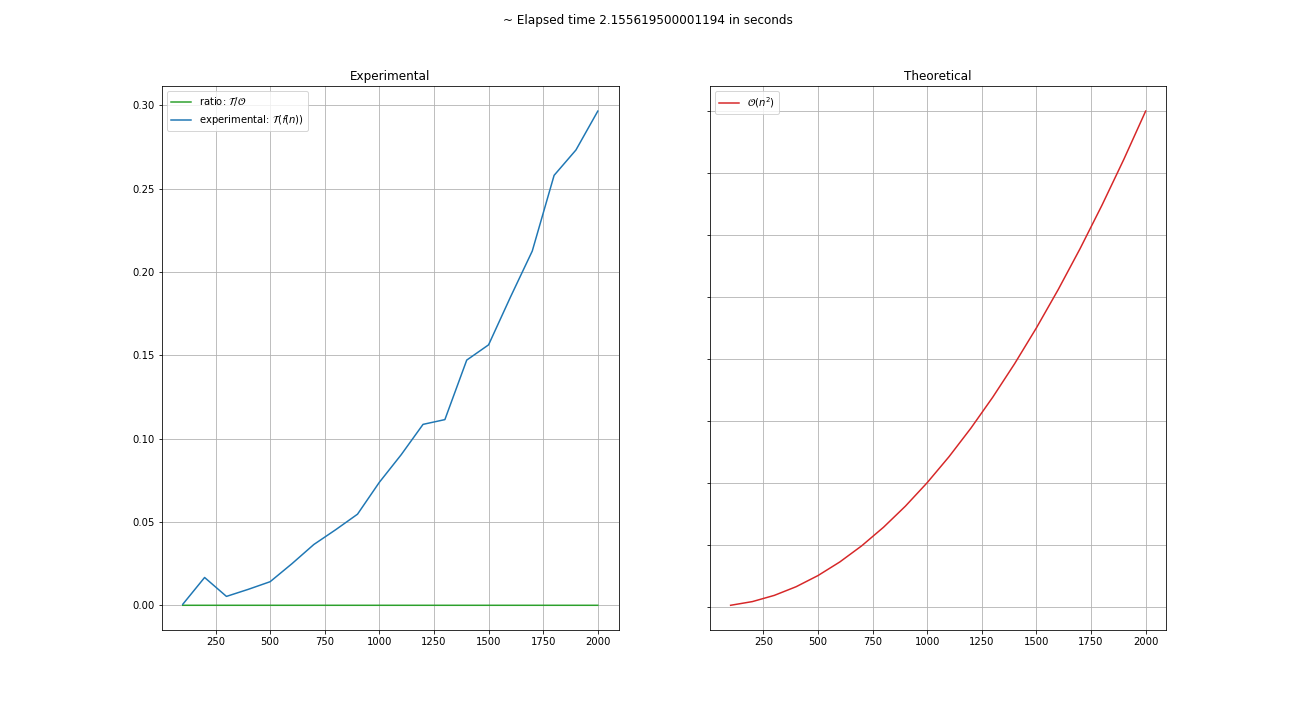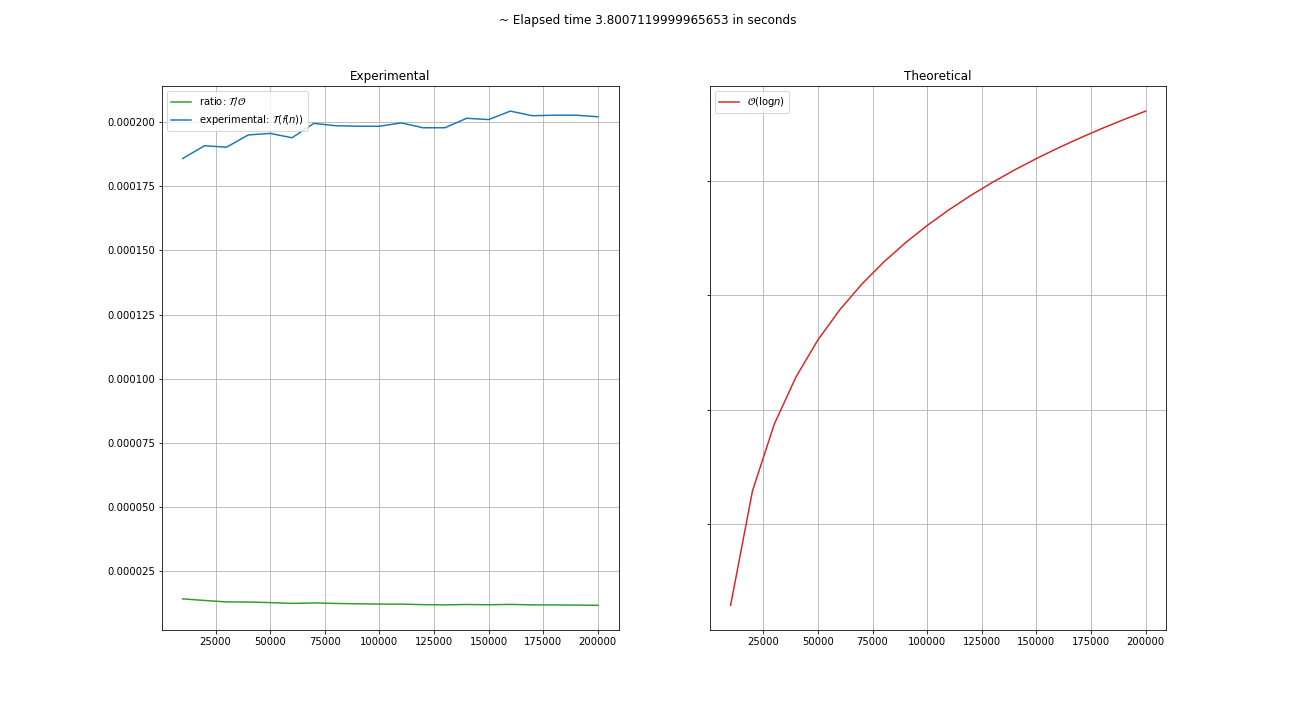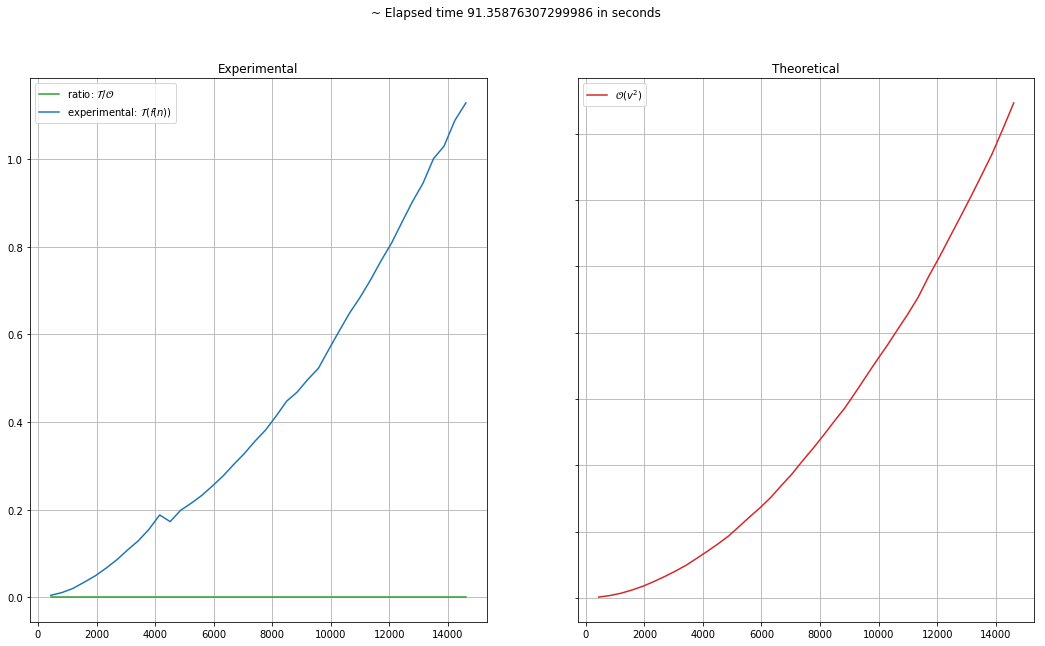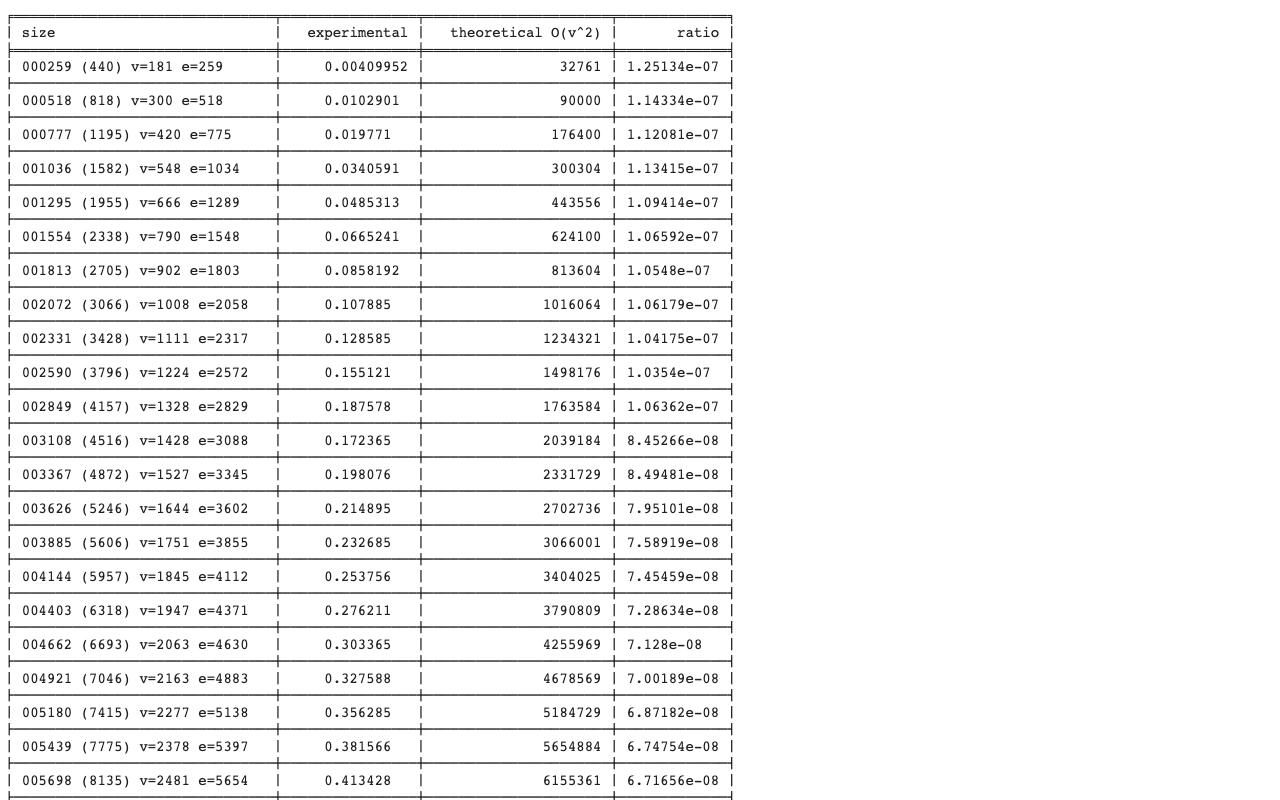A python library to measure algorithms execution time and compare with its theoretical complexity

# Almetro Library

version number: 1.0.7 author: Arnour Sabino

## Overview

A python library to measure algorithms execution time and compare with its theoretical complexity.## Installation / Usage

To install use pip:

$pip install almetro  Or clone the repo: $ git clone https://github.com/arnour/almetro.git
$python setup.py install  ## Information Almetro uses timeit module from python to time your algorithms. See more here ## Examples Applying Almetro to a quadratic algorithm: import almetro from almetro.algorithms import loop_n_quadratic from almetro.complexity import cn_quadratic from almetro.instance import growing metro = almetro\ .new()\ .with_execution(trials=5)\ .with_instances(instances=20, provider=growing(initial_size=100, growth_size=100))\ .metro(algorithm=loop_n_quadratic, complexity=cn_quadratic) chart = metro.chart() chart.show()Applying Almetro to a lg n algorithm: import almetro from almetro.algorithms import loop_n_log from almetro.complexity import clog_n from almetro.instance import growing metro = almetro\ .new()\ .with_execution(trials=100)\ .with_instances(instances=20, provider=growing(initial_size=10000, growth_size=10000))\ .metro(algorithm=loop_n_log, complexity=clog_n) chart = metro.chart() chart.show()Customazing execution: import almetro from almetro.complexity import Complexity from almetro.instance import generator my_custom_complexity = Complexity( theoretical=lambda v=1, e=1, c=1: v * v, experimental=lambda v=1, e=1, c=1: v + e, text='O(v^2)', latex=r'$\mathcal{O}(v^2)\$'
)

# You need to provide instances as dict: {'name': '', 'size': {}, 'value': {}}
# Size must contains all needed theoretical complexity arguments
# Value must contain all needed algorithms arguments

def my_custom_instances(n):
g = create_some_graph()
for _ in range(n):
yield {
'name': 'my instance name',
'size': {'v': len(g.nodes()), 'e': len(g.edges())}, 'c': some_order_value(),
'value': {
'graph': g,
'v': len(g.nodes())
}
}

def my_custom_algorithm(graph, v):
# Do some stuff
pass

N = 50

instances_generator = my_custom_instances(N)

# Trials determine how many times each instance will be repeated for Almetro to pick the min time.
metro = almetro\
.new()\
.with_execution(trials=5)\
.with_instances(instances=N, provider=generator(instances_generator)\
.metro(algorithm=my_custom_algorithm, complexity=my_custom_complexity)

metro.chart().show()metro.table().show()## Project details

This version1.0.71.0.61.0.51.0.41.0.31.0.21.0.11.0.0

Uploaded source
Uploaded py3